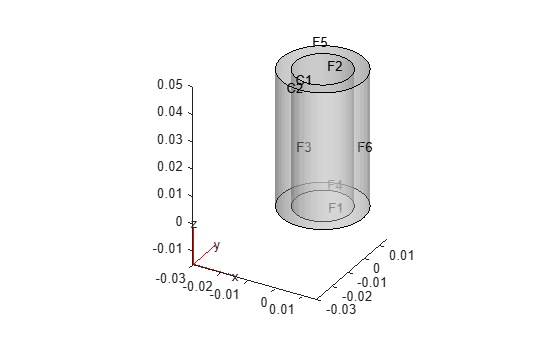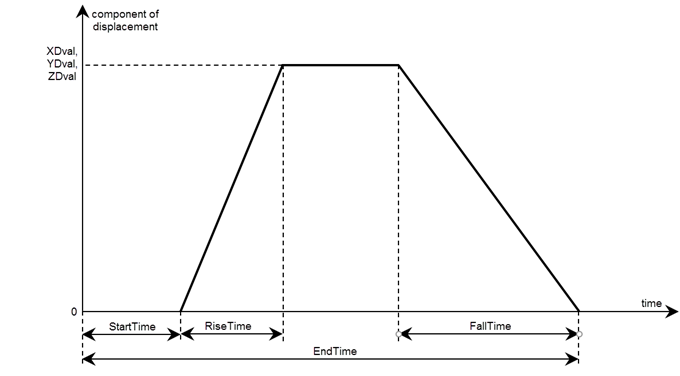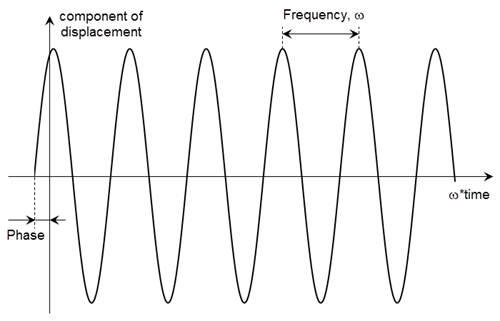Documentation

# structuralBC

Specify boundary conditions for structural model

## Syntax

``structuralBC(structuralmodel,RegionType,RegionID,'Constraint',Cval)``
``structuralBC(structuralmodel,RegionType,RegionID,'Displacement',Dval)``
``structuralBC(structuralmodel,RegionType,RegionID,'XDisplacement',XDval,'YDisplacement',YDval,'ZDisplacement',ZDval)``
``structuralBC(structuralmodel,RegionType,RegionID,'XDisplacement',XDval,Name,Value)``
``structuralBC(structuralmodel,RegionType,RegionID,'YDisplacement',YDval,Name,Value)``
``structuralBC(structuralmodel,RegionType,RegionID,'ZDisplacement',ZDval,Name,Value)``
``structuralBC(structuralmodel,RegionType,RegionID,'Constraint','multipoint')``
``structuralBC(structuralmodel,RegionType,RegionID,'Constraint','multipoint','Reference',Coords)``
``structuralBC(structuralmodel,RegionType,RegionID,'Constraint','multipoint','Reference',Coords,'Radius',R)``
``structuralBC(___,'Vectorized','on')``
``bc = structuralBC(___)``

## Description

example

````structuralBC(structuralmodel,RegionType,RegionID,'Constraint',Cval)` specifies one of the standard structural boundary constraints. Here, `Cval` can be `'fixed'`, `'free'`, `'roller'`, or `'symmetric'`. The default value is `'free'`.Avoid using `'symmetric'` for transient and modal analysis, since the symmetric constraint can prevent the participation of some structural modes.```

example

````structuralBC(structuralmodel,RegionType,RegionID,'Displacement',Dval)` enforces displacement on the boundary of type `RegionType` with `RegionID` ID numbers.```

example

````structuralBC(structuralmodel,RegionType,RegionID,'XDisplacement',XDval,'YDisplacement',YDval,'ZDisplacement',ZDval)` specifies the x-, y-, and z-components of the enforced displacement.`structuralBC` does not require you to specify all three components. Depending on your structural analysis problem, you can specify one or more components by picking the corresponding arguments and omitting others.```
````structuralBC(structuralmodel,RegionType,RegionID,'XDisplacement',XDval,Name,Value)`specifies the form and duration of the time-varying value of the x-component of the enforced displacement.```

example

````structuralBC(structuralmodel,RegionType,RegionID,'YDisplacement',YDval,Name,Value)` specifies the form and duration of the time-varying value of the y-component of the enforced displacement.```
````structuralBC(structuralmodel,RegionType,RegionID,'ZDisplacement',ZDval,Name,Value)` specifies the form and duration of the time-varying value of the z-component of the enforced displacement.```

example

````structuralBC(structuralmodel,RegionType,RegionID,'Constraint','multipoint')` sets the multipoint constraint using all degrees of freedom on the combination of geometric regions specified by `RegionType` and `RegionID`. The reference location for the constraint is the geometric center of all nodes on the combination of all specified geometric regions.This syntax is required if you intend to use results obtained with the model order reduction technique in the Simscape™ Multibody™ `Reduced Order Flexible Solid` block. Simscape models expect the connections at all joints to have six degrees of freedom, while Partial Differential Equation Toolbox™ uses two or three degrees of freedom at each node. Setting a multipoint constraint ensures that all nodes and all degrees of freedom for the specified geometric regions have a rigid constraint with the geometric center of all specified geometric regions altogether as the reference point. The reference location has six degrees of freedom.For better performance, specify geometric regions with a minimal number of nodes. For example, use a set of edges instead of using a face, and a set of vertices instead of using an edge.```
````structuralBC(structuralmodel,RegionType,RegionID,'Constraint','multipoint','Reference',Coords)` specifies the reference point for the multipoint constraint instead of using the geometric center of all specified regions as a reference point.```
````structuralBC(structuralmodel,RegionType,RegionID,'Constraint','multipoint','Reference',Coords,'Radius',R)` restricts the region for multipoint constraint to nodes within the circle (for a 2-D geometry) or sphere (for a 3-D geometry) of radius `R` around the reference point.```
````structuralBC(___,'Vectorized','on')` uses vectorized function evaluation when you pass a function handle as an argument. If your function handle computes in a vectorized fashion, then using this argument saves time. See Vectorization (MATLAB). For details on this evaluation, see Nonconstant Boundary Conditions.Use this syntax with any of the input arguments from previous syntaxes.```
````bc = structuralBC(___)` returns the structural boundary condition object using any of the input arguments from previous syntaxes.```

## Examples

collapse all

Apply fixed boundaries and traction on two ends of a bimetallic cable.

Create a structural model.

`structuralModel = createpde('structural','static-solid');`

Create nested cylinders to model a bimetallic cable.

`gm = multicylinder([0.01,0.015],0.05);`

Assign the geometry to the structural model and plot the geometry.

```structuralModel.Geometry = gm; pdegplot(structuralModel,'CellLabels','on','FaceLabels','on','FaceAlpha',0.4)```For each metal, specify the Young's modulus and Poisson's ratio.

```structuralProperties(structuralModel,'Cell',1,'YoungsModulus',110E9, ... 'PoissonsRatio',0.28); structuralProperties(structuralModel,'Cell',2,'YoungsModulus',210E9, ... 'PoissonsRatio',0.3);```

Specify that faces 1 and 4 are fixed boundaries.

`structuralBC(structuralModel,'Face',[1,4],'Constraint','fixed')`
```ans = StructuralBC with properties: RegionType: 'Face' RegionID: [1 4] Vectorized: 'off' Boundary Constraints and Enforced Displacements Displacement: [] XDisplacement: [] YDisplacement: [] ZDisplacement: [] Constraint: "fixed" Radius: [] Reference: [] Boundary Loads Force: [] SurfaceTraction: [] Pressure: [] TranslationalStiffness: [] ```

Specify the surface traction for faces 2 and 5.

`structuralBoundaryLoad(structuralModel,'Face',[2,5],'SurfaceTraction',[0;0;100])`
```ans = StructuralBC with properties: RegionType: 'Face' RegionID: [2 5] Vectorized: 'off' Boundary Constraints and Enforced Displacements Displacement: [] XDisplacement: [] YDisplacement: [] ZDisplacement: [] Constraint: [] Radius: [] Reference: [] Boundary Loads Force: [] SurfaceTraction: [3x1 double] Pressure: [] TranslationalStiffness: [] ```

Create a structural model.

`structuralModel = createpde('structural','static-solid');`

Create a block geometry.

`gm = multicuboid(0.2,0.1,0.05);`

Assign the geometry to the structural model and plot the geometry.

```structuralModel.Geometry = gm; pdegplot(structuralModel,'FaceLabels','on','FaceAlpha',0.5)```Specify the Young's modulus, Poisson's ratio, and mass density.

```structuralProperties(structuralModel,'YoungsModulus',74e9,... 'PoissonsRatio',0.42,... 'MassDensity',19.29e3);```

Specify the gravity load on the beam.

`structuralBodyLoad(structuralModel,'GravitationalAcceleration',[0;0;-9.8]);`

Specify that face 5 is a fixed boundary.

`structuralBC(structuralModel,'Face',5,'Constraint','fixed');`

Specify z-displacement on face 3 of the model. By leaving the x- and y-displacements unspecified, you enable face 3 to move in the x- and y-directions.

`structuralBC(structuralModel,'Face',3,'ZDisplacement',0.0001);`

Generate a mesh and solve the model.

```generateMesh(structuralModel); R = solve(structuralModel); ```

Plot the deformed shape with the x-component of normal stress.

`pdeplot3D(structuralModel,'ColorMapData',R.Stress.sxx,'Deformation',R.Displacement)`Now specify all three displacements on the same face. Here, the z-displacement is the same, but the x- and y-displacements are both zero. Face 3 cannot move in the x- and y-directions.

```structuralBC(structuralModel,'Face',3,'Displacement',[0;0;0.0001]); R = solve(structuralModel); pdeplot3D(structuralModel,'ColorMapData',R.Stress.sxx,'Deformation',R.Displacement)```Thus, specifying `'Displacement',[0;0;0.0001]` is equivalent to specifying `'XDisplacement',0,'YDisplacement',0,'ZDisplacement',0.0001`.

```structuralBC(structuralModel,'Face',3,'XDisplacement',0, ... 'YDisplacement',0, ... 'ZDisplacement',0.0001); R = solve(structuralModel); pdeplot3D(structuralModel,'ColorMapData',R.Stress.sxx,'Deformation',R.Displacement)```Use a function handle to specify a harmonically varying excitation in a beam.

Create a transient dynamic model for a 3-D problem.

`structuralmodel = createpde('structural','transient-solid');`

Create a geometry and include it in the model. Plot the geometry.

```gm = multicuboid(0.06,0.005,0.01); structuralmodel.Geometry = gm; pdegplot(structuralmodel,'FaceLabels','on','FaceAlpha',0.5) view(50,20)```Specify the Young's modulus, Poisson's ratio, and mass density of the material.

```structuralProperties(structuralmodel,'YoungsModulus',210E9, ... 'PoissonsRatio',0.3, ... 'MassDensity',7800);```

Fix one end of the beam.

`structuralBC(structuralmodel,'Face',5,'Constraint','fixed');`

Apply a sinusoidal displacement along the y-direction on the end opposite to the fixed end of the beam.

```yDisplacementFunc = @(location,state) ones(size(location.y))*1E-4*sin(50*state.time); structuralBC(structuralmodel,'Face',3,'YDisplacement',yDisplacementFunc)```
```ans = StructuralBC with properties: RegionType: 'Face' RegionID: 3 Vectorized: 'off' Boundary Constraints and Enforced Displacements Displacement: [] XDisplacement: [] YDisplacement: [function_handle] ZDisplacement: [] Constraint: [] Radius: [] Reference: [] Boundary Loads Force: [] SurfaceTraction: [] Pressure: [] TranslationalStiffness: [] Time Variation of Force, Pressure, or Enforced Displacement StartTime: [] EndTime: [] RiseTime: [] FallTime: [] Sinusoidal Variation of Force, Pressure, or Enforced Displacement Frequency: [] Phase: [] ```

Specify a harmonically varying excitation by specifying its frequency.

Create a transient dynamic model for a 3-D problem.

`structuralmodel = createpde('structural','transient-solid');`

Create a geometry and include it in the model. Plot the geometry.

```gm = multicuboid(0.06,0.005,0.01); structuralmodel.Geometry = gm; pdegplot(structuralmodel,'FaceLabels','on','FaceAlpha',0.5) view(50,20)```Specify the Young's modulus, Poisson's ratio, and mass density of the material.

```structuralProperties(structuralmodel,'YoungsModulus',210E9, ... 'PoissonsRatio',0.3, ... 'MassDensity',7800);```

Fix one end of the beam.

`structuralBC(structuralmodel,'Face',5,'Constraint','fixed');`

Apply a sinusoidal displacement along the y-direction on the end opposite to the fixed end of the beam.

`structuralBC(structuralmodel,'Face',3,'YDisplacement',1E-4,'Frequency',50);`

Fix one corner of a rectangular plate to restrain all rigid body motions of the model.

Create a structural model for static plane-stress analysis.

`model = createpde('structural','static-planestress'); `

Create the geometry and include it in the structural model.

```length = 1; width = 0.5; radius = 0.1; R1 = [3 4 -length length length -length ... -width -width width width]'; C1 = [1 0 0 radius 0 0 0 0 0 0]'; gdm = [R1 C1]; ns = char('R1','C1'); g = decsg(gdm,'R1- C1',ns'); geometryFromEdges(model,g);```

Plot the geometry, displaying edge labels.

```figure pdegplot(model,'EdgeLabels','on'); axis([-1.2*length 1.2*length ... -1.2*width 1.2*width])```Plot the geometry, displaying vertex labels.

```figure pdegplot(model,'VertexLabels','on'); axis([-1.2*length 1.2*length ... -1.2*width 1.2*width])```Specify the Young's modulus and Poisson's ratio of the material.

`structuralProperties(model,'YoungsModulus',210E9,'PoissonsRatio',0.3);`

Set the x-component of displacement on the left edge of the plate to zero to resist the applied load.

`structuralBC(model,'Edge',3,'XDisplacement',0);`

Apply the surface traction with a nonzero x-component on the right edge of the plate.

`structuralBoundaryLoad(model,'Edge',1,'SurfaceTraction',[100000 0]);`

Set the y-component of displacement at the bottom-left corner (vertex 3) to zero to restraint the rigid body motion.

`structuralBC(model,'Vertex',3,'YDisplacement',0);`

Generate the mesh, using `Hmax` to control the mesh size. A fine mesh lets you capture the gradation in the solution accurately.

`generateMesh(model,'Hmax',radius/6);`

Solve the problem.

`R = solve(model); `

Plot the `x`-component of the normal stress distribution.

```pdeplot(model,'XYData',R.Stress.sxx); axis equal colormap jet title 'Normal Stress Along x-Direction';```Set multipoint constraints on two opposite sides of a beam.

Create a transient structural model for a 3-D problem.

`structuralmodel = createpde('structural','transient-solid');`

Create a geometry and include it in the model. Plot the geometry.

```gm = multicuboid(0.1,0.01,0.01); structuralmodel.Geometry = gm; pdegplot(structuralmodel,'EdgeLabels','on','FaceAlpha',0.5)```Specify the Young's modulus, Poisson's ratio, and mass density of the material.

```structuralProperties(structuralmodel,'YoungsModulus',70E9, ... 'PoissonsRatio',0.3, ... 'MassDensity',2700);```

Generate a mesh.

`generateMesh(structuralmodel);`

Set the multipoint constraint on the right side of the beam. For better performance, set the constraint on the set of edges bounding the right side of the beam instead of setting it on the entire face.

`structuralBC(structuralmodel,'Edge',[4,6,9,10],'Constraint','multipoint')`
```ans = StructuralBC with properties: RegionType: 'Edge' RegionID: [4 6 9 10] Vectorized: 'off' Boundary Constraints and Enforced Displacements Displacement: [] XDisplacement: [] YDisplacement: [] ZDisplacement: [] Constraint: "multipoint" Radius: [] Reference: [] Boundary Loads Force: [] SurfaceTraction: [] Pressure: [] TranslationalStiffness: [] Time Variation of Force, Pressure, or Enforced Displacement StartTime: [] EndTime: [] RiseTime: [] FallTime: [] Sinusoidal Variation of Force, Pressure, or Enforced Displacement Frequency: [] Phase: [] ```

Using the same approach, set the multipoint constraint on the left side of the beam.

`structuralBC(structuralmodel,'Edge',[2,8,11,12],'Constraint','multipoint')`
```ans = StructuralBC with properties: RegionType: 'Edge' RegionID: [2 8 11 12] Vectorized: 'off' Boundary Constraints and Enforced Displacements Displacement: [] XDisplacement: [] YDisplacement: [] ZDisplacement: [] Constraint: "multipoint" Radius: [] Reference: [] Boundary Loads Force: [] SurfaceTraction: [] Pressure: [] TranslationalStiffness: [] Time Variation of Force, Pressure, or Enforced Displacement StartTime: [] EndTime: [] RiseTime: [] FallTime: [] Sinusoidal Variation of Force, Pressure, or Enforced Displacement Frequency: [] Phase: [] ```

Reduce the model to all modes in the frequency range `[-Inf,500000]` and the interface degrees of freedom.

`R = reduce(structuralmodel,'FrequencyRange',[-Inf,500000])`
```R = ReducedStructuralModel with properties: K: [34x34 double] M: [34x34 double] NumModes: 22 RetainedDoF: [1x144 double] ReferenceLocations: [3x2 double] Mesh: [1x1 FEMesh] ```

## Input Arguments

collapse all

Structural model, specified as a `StructuralModel` object. The model contains the geometry, mesh, structural properties of the material, body loads, boundary loads, and boundary conditions.

Example: ```structuralmodel = createpde('structural','transient-solid')```

Geometric region type, specified as `'Vertex'`, `'Edge'`, or, for a 3-D model, `'Face'`.

You cannot use the following geometric region types if you specify the `'roller'` or `'symmetric'` value for the boundary constraint `Cval`:

• `'Edge'` for a 3-D model

• `'Vertex'` for a 2-D or 3-D model

Example: `structuralBC(structuralmodel,'Face',[2,5],'XDisplacement',0.1)`

Data Types: `char` | `string`

Geometric region ID, specified as a vector of positive integers. Find the region IDs by using `pdegplot`.

Example: `structuralBC(structuralmodel,'Face',[2,5],'XDisplacement',0.01)`

Data Types: `double`

Enforced displacement, specified as a numeric vector or function handle. A numeric vector must contain two elements for a 2-D model and three elements for a 3-D model. The function must return a two-row matrix for a 2-D model and a three-row matrix for a 3-D model. Each column of the matrix must correspond to an enforced displacement vector at the boundary coordinates provided by the solver. In case of a transient structural model, `Dval` also can be a function of time.

Example: `structuralBC(structuralmodel,'Face',[2,5],'Displacement',[0;0;0.01])`

Data Types: `double` | `function_handle`

x-component of enforced displacement, specified as a number or function handle. The function must return a row vector. Each element of this vector corresponds to the x-component value of the enforced displacement at the boundary coordinates provided by the solver. In case of a transient structural model, `XDval` also can be a function of time.

Example: `structuralBC(structuralmodel,'Face',[2,5],'XDisplacement',0.01)`

Data Types: `double` | `function_handle`

y-component of enforced displacement, specified as a number or function handle. The function must return a row vector. Each element of this vector corresponds to the y-component value of the enforced displacement at the boundary coordinates provided by the solver. In case of a transient structural model, `YDval` also can be a function of time.

Example: `structuralBC(structuralmodel,'Face',[2,5],'YDisplacement',0.01)`

Data Types: `double` | `function_handle`

z-component of enforced displacement, specified as a number or function handle. The function must return a row vector. Each element of this vector corresponds to the z-component value of the enforced displacement at the boundary coordinates provided by the solver. In case of a transient structural model, `ZDval` also can be a function of time.

Example: `structuralBC(structuralmodel,'Face',[2,5],'ZDisplacement',0.01)`

Data Types: `double` | `function_handle`

Standard structural boundary constraints, specified as `'free'`,`'fixed'`,`'roller'`, `'symmetric'`, or `'MPC'`.

You cannot use the `'roller'` and `'symmetric'` values with the following geometric region types:

• `'Edge'` for a 3-D model

• `'Vertex'` for a 2-D or 3-D model

Example: `structuralBC(structuralmodel,'Face',[2,5],'Constraint','fixed')`

Data Types: `char` | `string`

Reference point location for the multipoint constraint, specified as a 2-by-1 (for a 2-D geometry) or 3-by-1 (for a 3-D geometry) numeric vector.

Example: `structuralBC(structuralmodel,'Vertex',[1,3,5:10],'Constraint','multipoint','Reference',[0;0;1])`

Data Types: `double`

Radius of a circle (for a 2-D geometry) or a sphere (for a 3-D geometry) around the reference point location for the multipoint constraint, specified as a positive number.

Example: `structuralBC(structuralmodel,'Vertex',[1,3,5:10],'Constraint','multipoint','Reference',[0;0;1],'Radius',0.2)`

Data Types: `double`

### Name-Value Pair Arguments

Example: `structuralBC(structuralmodel,'Face',[2,5],'XDisplacement',0.01,'RiseTime',0.5,'FallTime',0.5,'EndTime',3)`

Use one or more name-value pair arguments to specify the form and duration of the time-varying value of a component of displacement. Specify the displacement value using one of the following arguments: `XDval`, `YDval`, or `ZDval`. You cannot use these name-value pair arguments to specify more than one time-varying component or to specify the `Dval` value.

You can model rectangular, triangular, and trapezoidal displacement pulses. If the start time is 0, you do not need to specify it.

• For a rectangular pulse, specify the start and end times.

• For a triangular pulse, specify the start time and any two of the following times: rise time, fall time, and end time. You also can specify all three times, but they must be consistent.

• For a trapezoidal pulse, specify all four times.You can model a harmonic displacement by specifying its frequency and initial phase. If the initial phase is 0, you do not need to specify it.#### Rectangular, Triangular, or Trapezoidal Pulse

collapse all

Start time for the displacement component, specified as 0 or a positive number. Specify this argument only for transient structural models.

Example: `structuralBC(structuralmodel,'Face',[2,5],'XDisplacement',0.01,'StartTime',1,'EndTime',3)`

Data Types: `double`

End time for the displacement component, specified as a positive number equal or greater than the start time value. Specify this argument only for transient structural models.

Example: `structuralBC(structuralmodel,'Face',[2,5],'XDisplacement',0.01,'StartTime',1,'EndTime',3)`

Data Types: `double`

Rise time for the displacement component, specified as a positive number. Specify this argument only for transient structural models.

Example: `structuralBC(structuralmodel,'Face',[2,5],'XDisplacement',0.01,'RiseTime',0.5,'FallTime',0.5,'EndTime',3)`

Data Types: `double`

Fall time for the displacement component, specified as a positive number. Specify this argument only for transient structural models.

Example: `structuralBC(structuralmodel,'Face',[2,5],'XDisplacement',0.01,'RiseTime',0.5,'FallTime',0.5,'EndTime',3)`

Data Types: `double`

#### Harmonic Displacement

collapse all

Frequency of a sinusoidal displacement component value, specified as a positive number in radians per unit of time. Specify this argument only for transient structural models.

Example: `structuralBC(structuralmodel,'Face','XDisplacement',0.01,'Frequency',25)`

Data Types: `double`

Phase of a sinusoidal displacement component value, specified as a positive number in radians. Specify this argument only for transient structural models.

Example: `structuralBC(structuralmodel,'Face',[2,5],'XDisplacement',0.01,'Frequency',25,'Phase',pi/6)`

Data Types: `double`

## Output Arguments

collapse all

Handle to the boundary condition, returned as a `StructuralBC` object.

collapse all

### Degrees of Freedom (DoFs)

In Partial Differential Equation Toolbox, each node of a 2-D or 3-D geometry has two or three degrees of freedom (DoFs), respectively. DoFs correspond to translational displacements. If the number of mesh points in a model is `NumNodes`, then the toolbox assigns the IDs to the degrees of freedom as follows:

• Numbers from 1 to `NumNodes` correspond to an `x`-displacement at each node.

• Numbers from `NumNodes+1` to `2*NumNodes` correspond to a `y`-displacement at each node.

• Numbers from `2*NumNodes+1` to `3*NumNodes` correspond to a `z`-displacement at each node of a 3-D geometry.

## Tips

• Restrain all rigid body motions by specifying as many boundary conditions as needed. If you do not restrain all rigid body motions, the entire geometry can freely rotate or move. The resulting linear system of equations is singular. The system can take a long time to converge, or it might not converge at all. If the system converges, the solution includes a large rigid body motion in addition to deformation.

#### Introduced in R2017b

##### SupportGet trial now